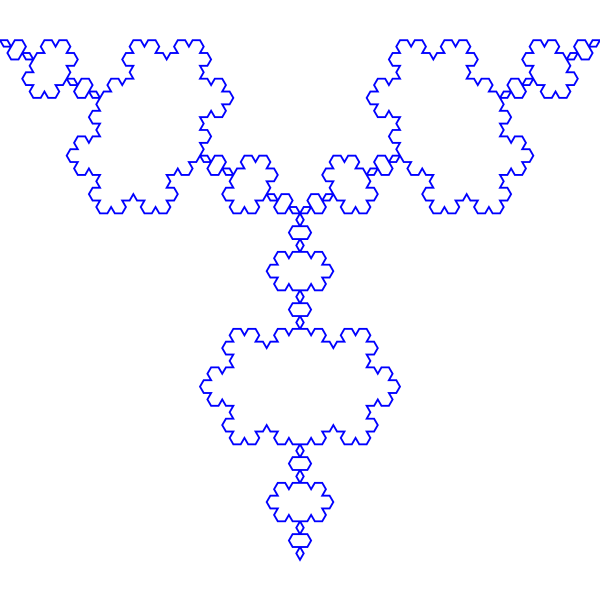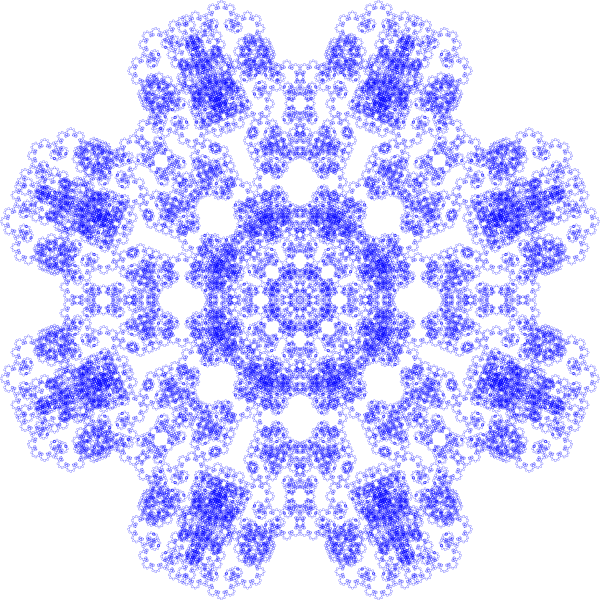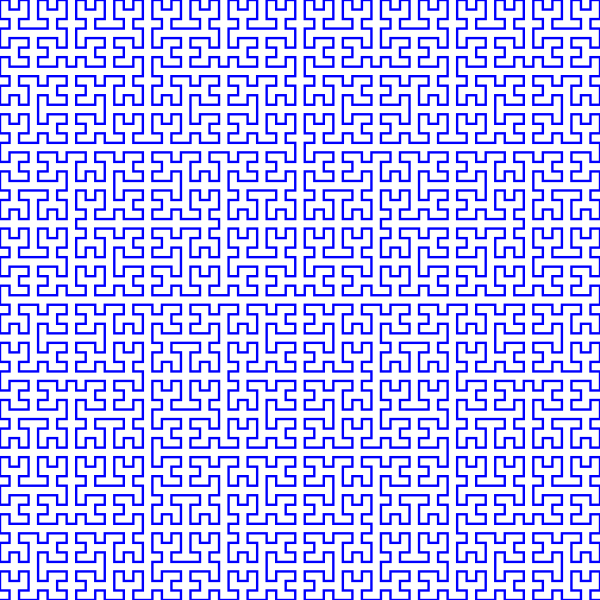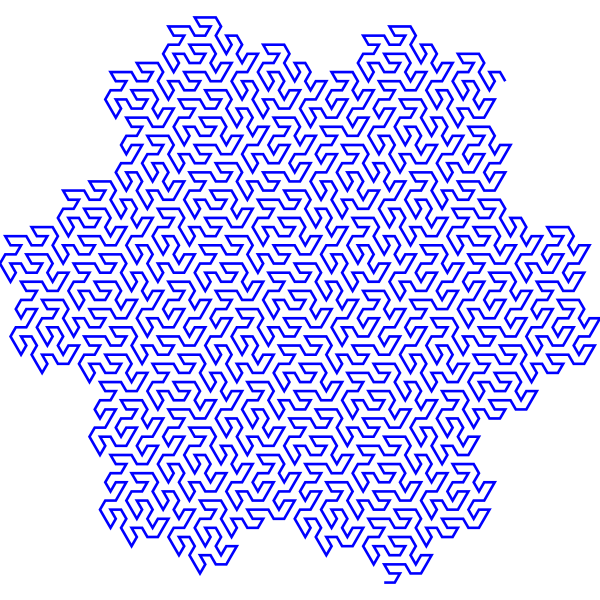Options
All
• Public
• Public/Protected
• All

#This project is part of the @thi.ng/umbrella monorepo.

Small, functional, highly customizable, iterator based L-System architecture for arbitrary rules, basic support for stochastic behaviors and with separation between symbol expansion and interpretation / execution. A base 2D turtle implementation is included. 0.6KB gzipped.

Partially based on Clojure version of @thi.ng/thingybot.

Planned features:

• parametric grammars
• max expansion length enforcement
• convergence testing
• 3D turtle implementation

### Status

BETA - possibly breaking changes forthcoming

Search or submit any issues for this package

## Installation

``````yarn add @thi.ng/lsys
``````
``````// ES module
<script type="module" src="https://unpkg.com/@thi.ng/lsys?module" crossorigin></script>

// UMD
<script src="https://unpkg.com/@thi.ng/lsys/lib/index.umd.js" crossorigin></script>
``````

Package sizes (gzipped, pre-treeshake): ESM: 679 bytes / CJS: 752 bytes / UMD: 868 bytes

## API

Generated API docs

Examples``````import * as lsys from "@thi.ng/lsys";
import * as g from "@thi.ng/geom";
import * as fs from "fs";

// example L-Systems shown above

const examples = [
{ rules: { s: "[f++f++f]", f: "f+f--f+f" }, delta: Math.PI / 3, iter: 5 },
{ rules: { s: "[f-f-f-f-f-f-f-f]", f: "f---f+f+f+f+f+f+f---f" }, delta: Math.PI / 4, iter: 6 },
{ rules: { s: "[x]", x: "-yf+xfx+fy-", y: "+xf-yfy-fx+" }, delta: Math.PI / 2, iter: 7 },
{ rules: { s: "[a]", a: "a-b--b+a++aa+b-", b: "+a-bb--b-a++a+b" }, delta: Math.PI / 3, iter: 5 }
];

const impl = lsys.TURTLE_IMPL_2D;

examples.forEach(({ rules, delta, iter }, i) =>
fs.writeFileSync(
`lsys-ex\${i}.svg`,
g.asSvg(
g.svgDoc(
{ stroke: "#00f", "stroke-width": 0.25, width: 600, height: 600 },
...lsys.interpret(
// create turtle instance with customized delta (rot angle)
lsys.turtle2d({ delta }),
// customize implementation to process syms "a" & "b" as "f"
{ ...impl, a: impl.f, b: impl.f },
// recursively expand start rule "s"
lsys.expand(rules, "s", iter)
//convert result paths to polylines for SVG export
).paths.map(g.polyline)
)
)
)
);
``````

### Stochastic behaviors

The built-in default turtle implementation supports some basic stochastic features, e.g. randomization of growth direction and stochastic branch termination. This enables the creation of more organic looking structures, like shown in the following example:``````import { XsAdd } from "@thi.ng/random";

lsys.interpret(
// create turtle instance with customized delta (rot angle)
lsys.turtle2d({
// initial movement step distance
step: 20,
// initial direction
theta: -PI / 2,
// rotation offset
delta: PI / 10,
// direction jitter (percentage of delta, i.e. here 50%)
jitter: 0.5,
// initial survival chance
aliveProb: 0.999,
// decay factors for rotation, step, branch survival chance
decayDelta: 0.98,
decayStep: 0.85,
decayAlive: 0.975,
// use seedable PRNG for deterministic outcome
}),
// process syms "a" & "g" as "f"
{ ...impl, a: impl.f, g: impl.f },
// recursively expand start rule "s" by ping-ponging between f & g
// (only difference between f & g is swapped branch orientations)
// see description of all symbols further below
lsys.expand(
{
s: "[f]",
f: "a[kp!>/-g]/a[kp!>/+g]",
g: "a[kp!>/+f]/a[kp!>/-f]"
},
"s",
13
)
)
``````

## Default turtle

### Options

The `turtle2d()` function creates a new state object for the L-System interpreter (`interpret()`). The initial state can be customized by providing a config object with the following options:

``````/**
* Current position
*/
pos: Vec;
/**
*/
theta: number;
/**
* Rotation angle for "+" / "-" symbols
*/
delta: number;
/**
* Max. random direction change when processing "/" symbol.
* Normalized percentage of `delta`. Default: 0.25 (25%)
*/
jitter: number;
/**
* Step distance. Default: 1
*/
step: number;
/**
* Probability to keep current branch alive when processing "k"
* symbol. Default: 0.99
*/
aliveProb: number;
/**
* Decay factor for `delta`. Should be in (0,1) interval.
* Default: 0.9
*/
decayDelta: number;
/**
* Decay factor for `step`. Should be in (0,1) interval.
* Default: 0.9
*/
decayStep: number;
/**
* Decay factor for `aliveProp`.
* Default: 0.95
*/
decayAlive: number;
/**
* PRNG to use for probability checks. Default: SYSTEM
*/
rnd: IRandom;
``````

### Symbols

• `f` - move forward & add to current path
• `g` - move forward & start new path
• `+` - rotate ccw
• `-` - rotate cw
• `>` - shrink rotation angle offset
• `<` - grow rotation angle offset
• `/` - jitter direction
• `k` - stochastically kill branch
• `p` - decay survival chance
• `P` - increase survival chance
• `!` - decay step distance
• `^` - grow step distance
• `[` - start branch / store context on stack
• `]` - end branch / pop context from stack

## Authors

Karsten Schmidt

If this project contributes to an academic publication, please cite it as:

``````@misc{thing-lsys,
title = "@thi.ng/lsys",
author = "Karsten Schmidt",
note = "https://thi.ng/lsys",
year = 2019
}
``````